# Samacheer Kalvi 9th Maths Book Solutions Chapter 4 | Geometry Answers For Tamil Nadu Board

Samacheer Kalvi 9th Maths Book Solutions Chapter 4 – Geometry is available here. The Samacheer Kalvi 9th Maths book answers of Chapter 4, available at BYJU’S, contain step by step explanations designed by our mathematics experts. All these important questions are based on the new pattern prescribed by the Tamil Nadu board. Students can also get the solutions of other chapters on Samacheer Kalvi 9th Maths solutions. One of the best resources to master the subject and ace the exams is the Samacheer Kalvi Class 9 Maths Chapter 4 Questions and Solutions.

## Samacheer Kalvi Class 9 Maths Chapter 4 Questions with Solutions

Chapter 4 of the Samacheer Kalvi 9th Maths guide will help the students to solve problems related to the types of angles, transversal, triangles, types of triangles, quadrilaterals, their properties and types, parts of a circle and their properties, cyclic quadrilaterals, practical geometry.

### Samacheer Kalvi 9th Maths Chapter 4: Geometry Book Exercise 4.1 Questions and Solutions

Question 1: In the figure, AB is parallel to CD, find x.

Solution:

(i) From the figure,

∠1 = 140° (corresponding angles are equal)

∠2 = 40o (∠A + ∠2 = 180°)

∠3 = 30° (∠3 + 150o = 180°)

∠4 = 110° (∠2 + ∠3 + ∠4 = 180°)

∠x = 70o (∠4 + ∠x = 180o)

(ii) From the figure,

∠1 = 48°

∠3 =108° (∠1 + 24° + ∠3 = 180°)

∠4 =108° (If two lines are intersect, then the vertically the opposite angles are equal)

∠5 = 72° (∠3 + ∠5 =180°)

∠3 + ∠4 + ∠5 = 108° + 108° + 72°

∠x = 288°

(iii) From the figure,

∠D = 53° 1… (∠B and ∠D are alternate interior angles)

Sum of the three angles of a triangle is 180°

∠x = 180°- (38°+ 53°)

= 180°- 91°

= 89°

Question 2: The angles of a triangle are in the ratio 1: 2 : 3, find the measure of each angle of the triangle.

Solution:

Let the angles be x, 2x and 3x.

Sum of the three angles of a triangle 180°.

x + 2x + 3x = 180°

6x = 180°

x = 180o / 6

x = 30o

2x = 2 * 30 = 60°

3x = 3 * 30 = 90°

The 3 angles of the triangle are 30°, 60°, 90°.

Question 3: Consider the given pairs of triangles and say whether each pair is that of congruent triangles. If the triangles are congruent, say ‘how’; if they are not congruent say ‘why’ and also say if a small modification would make them congruent: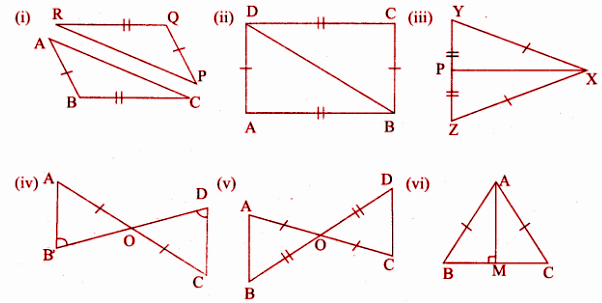Solution:

(i) Consider △PQR and △ABC

Given:

RQ = BC

PQ = AB

△ABC is not congruent to △PQR

If PR = AC, then △ABC ⩭ △PQR

(ii) Consider △ABD and △BCD for the triangles to be congruent.

Given:

AB = DC

AD = BC and AB is the common side.

By the SSS [three sides being equal] rule, △ABD ⩭ △BCD.

(iii) Consider △PXY and △PXZ

Given:

XY = XZ

PY = PZ and PX is common.

By SSS rule, △PXY ⩭ △PXZ.

(iv) Consider △OAB and △ODC

Given:

OA = OC

∠ABO = ∠ODC and ∠AOB = ∠DOC [vertically opposite angles]

By AAS rule, △OAB = △ODC.

(v) Consider △AOB and △DOC

Given:

AO = OC

OB = OD and ∠AOB = ∠DOC [vertically opposite angles]

By SAS rule, △AOB = △DOC.

(vi) Consider △AMB and △AMC

Given:

AB = AC

∠AMB = ∠AMC = 90o

AM is common.

By RHS rule, △AMB ⩭ △AMC.

Question 4: ΔABC and ΔDEF are two triangles in which AB = DF, ∠ACB = 70°, ∠ABC = 60°; ∠DEF = 70° and ∠EDF = 60°. Prove that the triangles are congruent.

Solution:

In ΔABC, ∠ACB = 70°, ∠ABC = 60°

∠BAC = 180°- (70°+ 60°)

= 180° – 130°

= 50°

In ΔDEF, ∠DEF = 70°, ∠EDF = 60°

∠DFE = 180° – (70° + 60°)

= 180° – 130°

= 50°

∠A = ∠F

AB = DF and

∠B = ∠D

By ASA rule ΔABC ⩭ ΔFDE

Question 5: Find all the three angles of the ΔABC.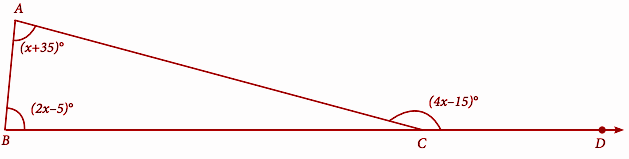Solution:

Exterior angle = Sum of the two opposite interior angles.

4x – 15 = 2x – 5 + x + 35

4x = 3x + 30 + 15

4x – 3x = 45°

x = 45°

∠A = x + 35

= 45° + 35°

= 80°

∠B = 2x – 5

= 2(45°) – 5

= 90 – 5°

= 85°

∠C = 4x – 15

= 4(45) – 15°

= 180° – 15°

= 165°

### Samacheer Kalvi 9th Maths Chapter 4: Geometry Book Exercise 4.2 Questions and Solutions

Question 1: The angles of a quadrilateral are in the ratio 2:4:5:7. Find all the angles.

Solution:

Let the four angles be 2x, 4x, 5x and 7x.

Sum of interior angles of quadrilaterals = 360o

2x + 4x + 5x + 7x = 360o

18x = 360o

x = 360 / 18 = 20

x = 20

2x = 2(20) = 40

4x = 4(20) = 80

5x = 5(20) = 100

7x = 7(20) = 140

The required angles are 40, 80, 100 and 140.

Question 2: In a quadrilateral ABCD, ∠A = 72° and ∠C is the supplementary of ∠A. The other two angles are 2x -10 and x + 4. Find the value of x and the measure of all the angles.

Solution:

∠A + ∠C = 180o

72 + ∠C = 180o

∠C = 180 – 72

∠C = 108

∠B + ∠D = 180

2x – 10 + x + 4 = 180

3x – 6 = 180

3x = 186

x = 186 / 3

x = 62

2x – 10 = 2(62) – 10

= 124 – 10

= 114

x + 4 = 62 + 4 = 66

The required angles are 72, 108, 114 and 66.

Question 3: ABCD is a rectangle whose diagonals AC and BD intersect at O. If ∠OAB = 46°, find ∠OBC.

Solution:

Each angle in a rectangle is 90o.

The diagonals of a rectangle cut each other into their half.

So,

AO = BO

∠OBA = ∠OAB

∠OBA = 46° ——- (1)

∠ABC = ∠OBA + ∠OBC

90° = ∠46° + ∠OBC

∠OBC = 44°

Question 4: The lengths of the diagonals of a Rhombus are 12 cm and 16 cm. Find the side of the rhombus.

Solution:

In a rhombus, the diagonals cut each other at right angles.

In triangle OAB,

AB2 = OA2 + OB2

AB2 = 62 + 82

AB2 = 36 + 64

AB2 = 100

On taking square root on both sides,

AB = 10

The side length of a rhombus is 10 cm.

Question 5: Show that the bisectors of angles of a parallelogram form a rectangle.

Solution: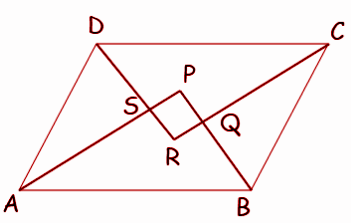∠SAD = (1 / 2) ∠A —-(1)

∠ADS = (1 / 2) ∠D —-(2)

(1) + (2)

∠SAD + ∠ASD + ∠SDA = 180

∠SAD + ∠SDA + ∠ASD = 180

90 + ∠ASD = 180

∠ASD = 180 – 90

∠ASD = 90, ∠PSR = 90o (Vertically opposite angle)

Hence PQRS is a rectangle.

Question 6: If a triangle and a parallelogram lie on the same base and between the same parallels, then prove that the area of the triangle is equal to half of the area of the parallelogram.

Solution: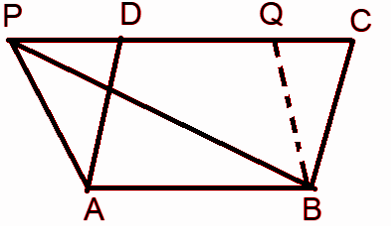Let ΔABP and a parallelogram ABCD be on the same base AB and between the same parallels AB and PC.

Prove that area of ΔPAB = (1 / 2) area of ABCD

Draw BQ ||AP to get another parallelogram. ABQP and ABCD lie on the same base AB and between the same parallels AB and PC.

Therefore, the area of ABQP = area of ABCD

ΔPAB ≅ ΔBQP (Diagonals PB divides the parallelogram ABQP into two congruent triangles).

Hence, the area of ΔPAB = area of ΔBQP ———–(2)

Area of ΔPAB = (1 / 2) area of trapezium ABQP —————–(3)

Thus, area of ΔPAB) = (1 / 2) area of ABCD.

Question 7: Iron rods a, b, c, d, e, and f are making a design in a bridge as shown in the figure. If a || b, c || d, e || f, find the marked angles between

(i) b and c

(ii) d and e

(iii d and f

(iv) c and f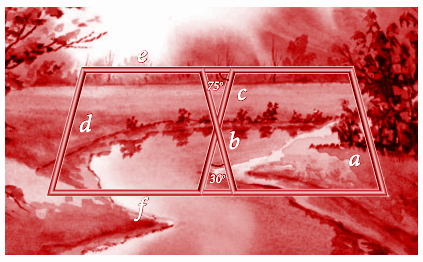Solution:

(i) The angle between b and c is 30o.

(ii) The angle between d and e :

75o + angle between d and e = 180o

The angle between d and e = 180 – 75 = 105o

(iii) The angle between d and f :

In a parallelogram, opposite angles will be equal.

The angle between d and is 75o.

(iv) The angle between c and f :

In a parallelogram, opposite angles will be equal.

The angle between c and f is 105o.

Question 8: In the given figure, ∠A = 64° , ∠ABC = 58°. If BO and CO are the bisectors of ∠ABC and ∠ACB respectively of ΔABC, find x° and y°.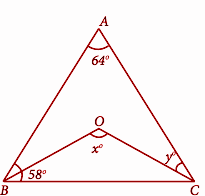Solution:

BO and CO are bisectors of ABC and ACB.

∠OBC = 58 / 2 = 29

In ΔABC,

∠ABC + ∠BAC + ∠BCA = 180o

58 + 64 + ∠BCA = 180o

∠BCA = 180 – 122

∠BCA = 58o

∠y = 58o

In ΔOBC,

∠OBC + ∠BOC + ∠BCO = 180o

29 + ∠BOC + 29 = 180o

∠BOC = 180 – 58

x = ∠BOC = 122o

Question 9: In the given figure, if AB = 2, BC = 6, AE = 6, BF = 8, CE = 7, and CF = 7, compute the ratio of the area of quadrilateral ABDE to the area of ΔCDF.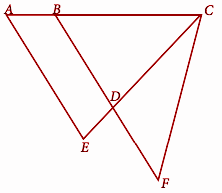Solution:

There are three triangles, Δ AEC, Δ BCF and Δ BDC.

Δ BCD is similar to ΔACE.

In ΔAEC,

AC = AB + BC = 2 + 6 = 8 cm

AE = 6 cm and EC = 7 cm

a = 8, b = 6 and c = 7

Using a formula for the area of a scalene triangle

= √s(s – a) (s – b) (s – c)

= √10.5(2.5) (4.5) (3.5)

= √413.43

= 20.33 —-(1)

In ΔBFC

BF = 8 cm , BC = 6 cm, and CF = 7 cm

s = (8 + 6 + 7) / 2 = 10.5

= √s(s – a) (s – b) (s – c)

= √10.5(2.5) (4.5) (3.5)

= √413.43

= 20.33 —-(1)

ΔBCD is similar to ΔACE and ΔBCF

To obtain the lengths of BD and CD for ΔBCD, use the linear scale factor.

8 / 6 = 4 / 3

8 (BF) / BD = 4 / 3

24 = 4BD

BD = 24 / 4 = 6

CE / CD = 8 / 6

7 / (CD) = 8 / 6

42 = 8 * CD

CD = 42 / 8 = 5.25

Area of ΔBCD :

S = (6 + 6 + 5.25) / 2 = 8.625

A = √8.625 (8.625 – 6) (8.625 – 6) (8.625 – 5.25)

= √200.58

= 14.16

Area of ΔCDF :

S = (5.25 + 7 + 2) / 2 = 7.125

A = √7.125(7.125 – 5.25) (7.125 – 7) (7.125 – 2)

= √8.558 = 2.925

= 2.925

Area of the trapezium ABDE = 20.33 – 14.16 = 6.17

The ratio of ABDE:ΔCDF

= 6.17 : 2.925

= 6 : 3 (approximately)

= 2 : 1

Question 10: In the figure, ABCD is a rectangle and EFGH is a parallelogram. Using the measurements given in the figure, what is the length d of the segment that is perpendicular to HE and FG?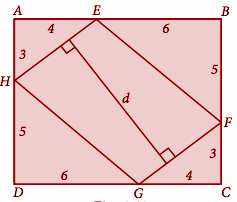Solution:

Area of ABCD = length x breadth

= DC * BC

= 10 x 8

= 80

Area of ΔAEH = Area of ΔCGF [As they are congruent by RHS rule]

Similarly, area of ΔBEF = Area of ΔDGH

Area of parallelogram = EFGH = Area of rectangle ABCD – 2 (area of ΔAEH) – 2 (area of ΔBEF)

= 80 – 2 (0.5 * 4 * 3) – 2 (0.5 * 6 * 5)

Area of parallelogram EFGH = 38

GE * d = 38

5 * d = 38 [In ΔGCF, GF2 = FC2 + GC2]

GF2 = 42 + 32

= 16 + 9

= 25

GF = 5

d = 38 / 5 = 7.6

Question 11: In parallelogram ABCD of the accompanying diagram, line DP is drawn bisecting BC at N and meeting AB (extended) at P. From vertex C, line CQ is drawn bisecting side AD at M and meeting AB (extended) at Q. Lines DP and CQ meet at O. Show that the area of triangle QPO is 9 / 8 of the area of the parallelogram ABCD.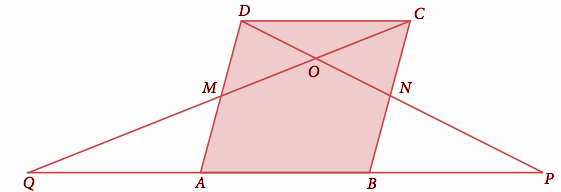Solution:

QPO = QAM + PBM + AMONB —–(1)

QPO = AMONB + MDC + NCD —–(2)

From (2),

= AMONB + MDC + NOC + DOC

= ABCD + DOC

Let ABCD = K

= K + DOC

DCNM is also a parallelogram.

So,

DOC = (¼) DCNM , 2 * ABCD = DCNM

= (1 / 8) * ABCD

= (k / 8)

QPO = K + [K / 8]

QPO = 9k / 8

### Samacheer Kalvi 9th Maths Chapter 4: Geometry Book Exercise 4.3 Questions and Solutions

Question 1: The diameter of the circle is 52cm and the length of one of its chords is 20cm. Find the distance of the chord from the centre.

Solution: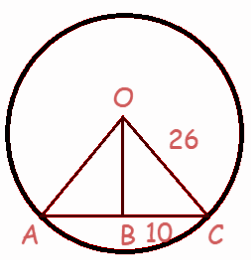Diameter = 52 cm

Radius of circle = 26 cm

In ΔBOC,

OC2 = OB2 + BC2

262 = OB2 + 102

676 – 100 = OB2

OB = √576

OB = 24

Question 2: The chord of length 30 cm is drawn at the distance of 8cm from the centre of the circle. Find the radius of the circle.

Solution: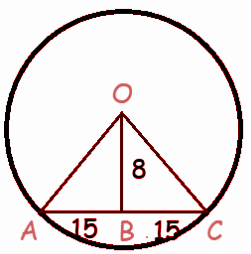In ΔBOC,

OC2 = OB2 + BC2

OC2 = 82 + 152

OC2 = 64 + 225

OC = √289

OC = 17

Question 3: Find the length of the chord AC where AB and CD are the two diameters perpendicular to each other of a circle with radius 4√2 cm and also find ∠OAC and ∠OCA.

Solution: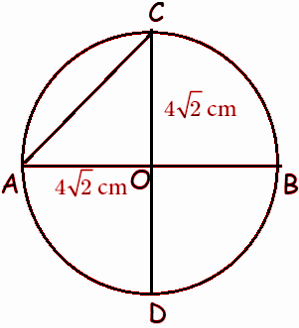In ΔAOC,

AC2 = AO2 + CO2

AC2 = (4√2)2 + (4√2)2

AC2 = 16(2) + 16(2)

AC2 = 64

AC = √64

AC = 8 cm

In triangle AOC,

COA = 90

OC = OA

Equal sides will form an equal angle.

OCA = OBC

Question 4: A chord is 12cm away from the centre of the circle of radius 15cm. Find the length of the chord.

Solution: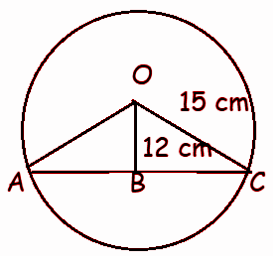Let the length of the chord BC be x.

In ΔBOC,

OC2 = OB2 + BC2

152 = 122 + x2

225 – 144 = x2

x = √81

x = 9 cm

AC = 2BC = 2(9) = 18 cm

Question 5: In a circle, AB and CD are two parallel chords with centre O and radius 10 cm such that AB = 16 cm and CD = 12 cm determine the distance between the two chords?

Solution:In ΔOPB,

OB2 = OP2 + PB2

OA = OB = OC = OD = 10 (Radius)

102 = OP2 + 82

OP2 = 100 – 64

OP2 = 36

OP = √36

OP = 6 cm

In ΔOQD,

OD2 = OQ2 + QD2

102 = OQ2 + 62

OQ2 = 100 – 36

OQ2 = 64

OQ = √64

OQ = 8 cm

Distance between two chords PQ = OQ – OP

= 8 – 6

= 2 cm

Question 6: Two circles of radii 5 cm and 3 cm intersect at two points and the distance between their centres is 4 cm. Find the length of the common chord.

Solution: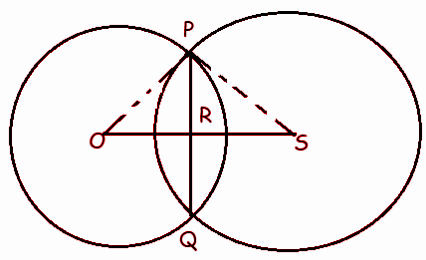OP = 3 cm, PS = 5 cm and OS = 4 cm

Let the length of the common chord RS be x.

OR = 4 – x

In ΔPOR,

OP2 = OR2 + PR2

32 = x2 + PR2

PR2 = 9 – x2 ——(1)

In ΔPRS,

PS2 = PR2 + RS2

52 = PR2 + (4 – x)2

PR2 = 25 – (4 – x)2

PR2 = 25 – (16 – 8x + x2)

PR2 = 25 – 16 + 8x – x2

PR2 = 9 + 8x – x2 —-(2)

(1) = (2)

9 – x2 = 9 + 8x – x2

8x = 0

x = 0

By applying the value of x in (1), we get

PR2 = 9 – 0

PR2 = 9

=> PR = 3

PQ = 2PR

PQ = 2(3)

PQ = 6 cm

Question 7: Find the value of x° in the following figures: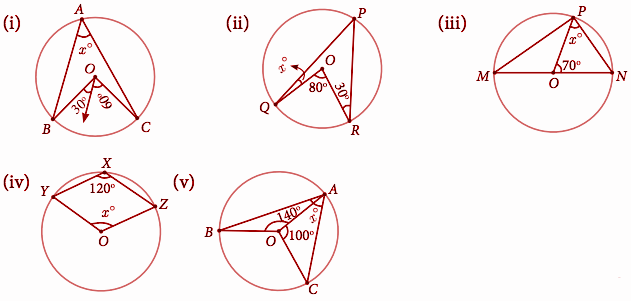Solution:

(i) ∠BOC = 2∠BAC

90° = 2x°

90 = 2x

Divide each side by 2.

45o = x

Equal sides will form equal angles.

∠OQP = ∠QPO = x°

∠ORP = ∠OPR = 30°

∠QPR = x° + 30°

2∠QPR = ∠QOR

2 (x° + 30°) = 80°

2 (x + 30) = 80

Divide each side by 2.

x + 30 = 40

x = 10

(iii) In ΔPON,

OP = ON (Radius) = x

∠ONP + ∠NOP + ∠OPN = 180

x° + 70° + x° = 180°

x + 70 + x = 180

2x + 70 = 180

Subtract 70 from each side.

2x = 110

Divide each side by 2.

x = 55o

(iv) x° = 120°

Equal sides will form equal angles.

∠OAC = ∠OCA = x°

In ΔOAC,

x° + x° + 100° = 180°

x + x + 100 = 180

2x + 100 = 180

Subtract 100 from each side.

2x = 80o

Divide each side by 2.

x = 40o

Question 8: In the given figure, ∠CAB = 25°, find ∠BDC, ∠DBA and ∠COB.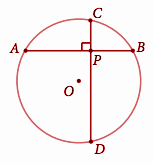Solution: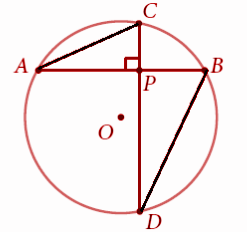In ΔAPC,

∠APC + ∠PCA + ∠CAP = 180°

90° + ∠PCA + 25° = 180°

∠PCA + 115° = 180°

Subtract 115° from each side.

∠PCA = 65°

∠DBA = 65° (Angle in the same segment)

∠BDC = 25° (angle in same segment)

∠COB = 50°